# 5 2 Solving Quadratic Equations Algebra 2 Learning

• Slides: 205. 2 Solving Quadratic Equations Algebra 2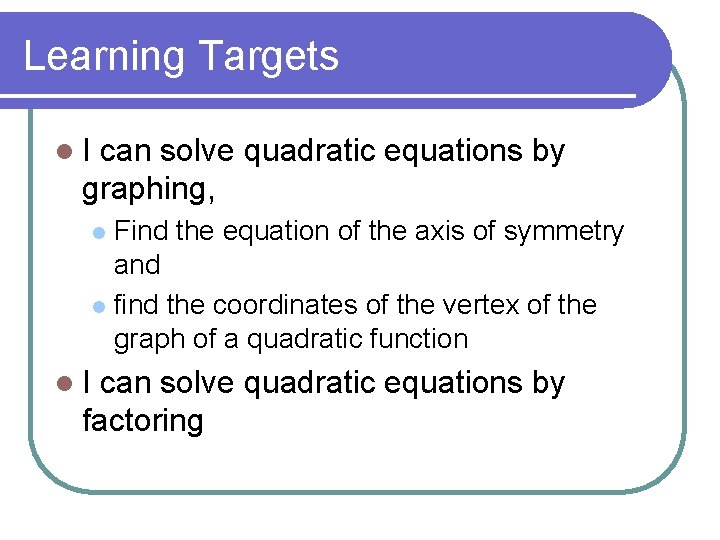Learning Targets l. I can solve quadratic equations by graphing, Find the equation of the axis of symmetry and l find the coordinates of the vertex of the graph of a quadratic function l l. I can solve quadratic equations by factoring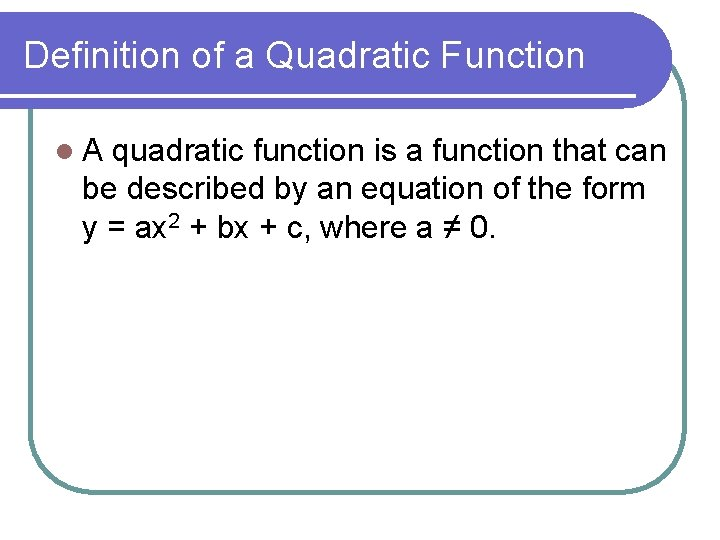Definition of a Quadratic Function l. A quadratic function is a function that can be described by an equation of the form y = ax 2 + bx + c, where a ≠ 0.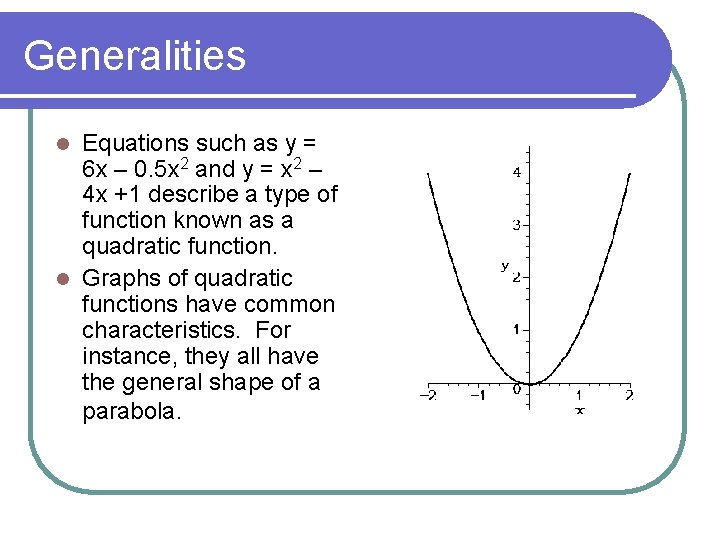Generalities Equations such as y = 6 x – 0. 5 x 2 and y = x 2 – 4 x +1 describe a type of function known as a quadratic function. l Graphs of quadratic functions have common characteristics. For instance, they all have the general shape of a parabola. lGeneralities The table and graph can be used to illustrate other common characteristics of quadratic functions. Notice the matching values in the y-column of the table. x x 2 – 4 x + 1 y -1 (-1)2 – 4(1) + 1 6 0 (0)2 – 4(0) + 1 1 1 (1)2 – 4(1) + 1 -2 2 (2)2 – 4(2) + 1 -3 3 (3)2 – 4(3) + 1 -2 4 (4)2 – 4(4) + 1 1 5 (5)2 – 4(5) + 1 6 y = x 2 – 4 x + 1 x=2 (2, -3)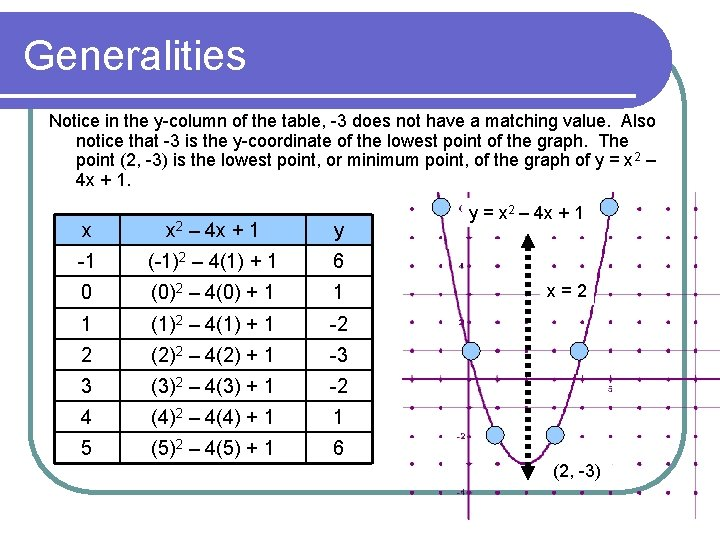Generalities Notice in the y-column of the table, -3 does not have a matching value. Also notice that -3 is the y-coordinate of the lowest point of the graph. The point (2, -3) is the lowest point, or minimum point, of the graph of y = x 2 – 4 x + 1. x x 2 – 4 x + 1 y -1 (-1)2 – 4(1) + 1 6 0 (0)2 – 4(0) + 1 1 1 (1)2 – 4(1) + 1 -2 2 (2)2 – 4(2) + 1 -3 3 (3)2 – 4(3) + 1 -2 4 (4)2 – 4(4) + 1 1 5 (5)2 – 4(5) + 1 6 y = x 2 – 4 x + 1 x=2 (2, -3)Maximum/minimum points l For the graph of y = 6 x – 0. 5 x 2, the point (6, 18) is the highest point, or maximum point. The maximum point or minimum point of a parabola is also called the vertex of the parabola. l The graph of a quadratic function will have a minimum point or a maximum, BUT NOT BOTH!!!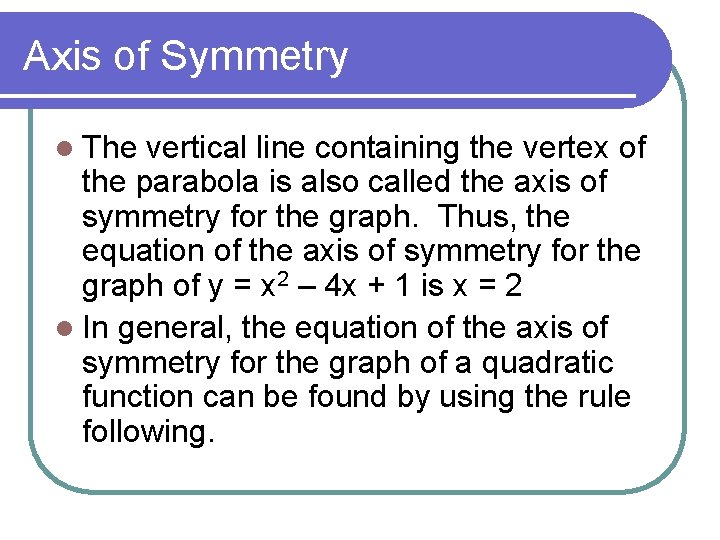Axis of Symmetry l The vertical line containing the vertex of the parabola is also called the axis of symmetry for the graph. Thus, the equation of the axis of symmetry for the graph of y = x 2 – 4 x + 1 is x = 2 l In general, the equation of the axis of symmetry for the graph of a quadratic function can be found by using the rule following.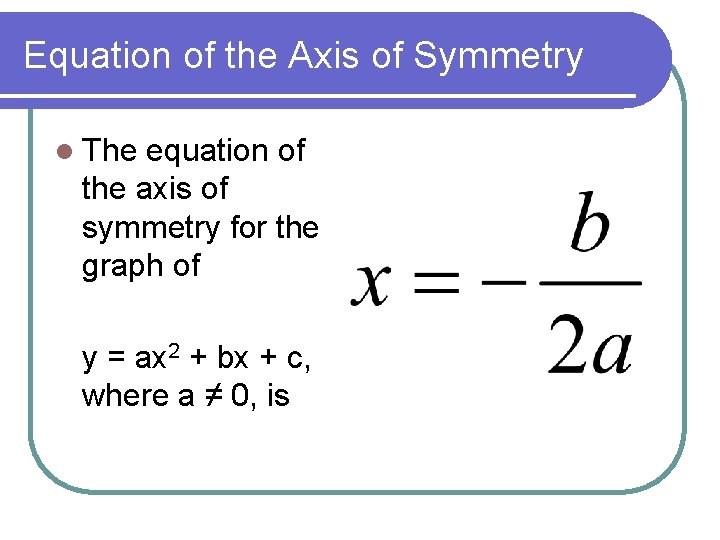Equation of the Axis of Symmetry l The equation of the axis of symmetry for the graph of y = ax 2 + bx + c, where a ≠ 0, is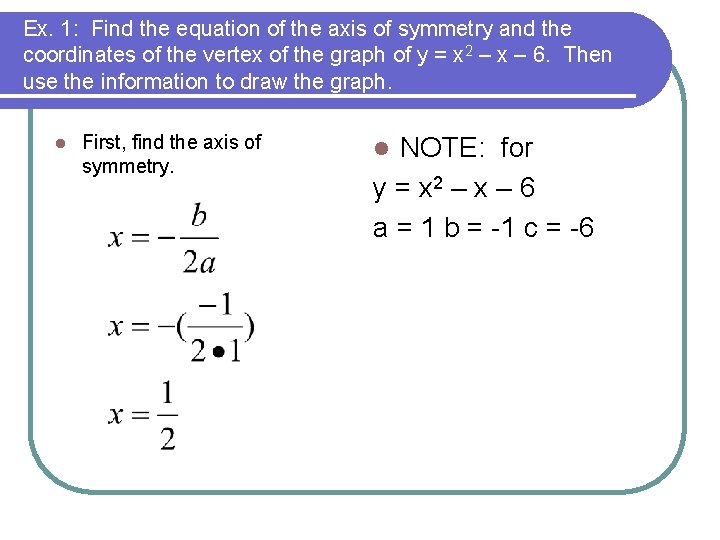Ex. 1: Find the equation of the axis of symmetry and the coordinates of the vertex of the graph of y = x 2 – x – 6. Then use the information to draw the graph. l First, find the axis of symmetry. NOTE: for y = x 2 – x – 6 a = 1 b = -1 c = -6 l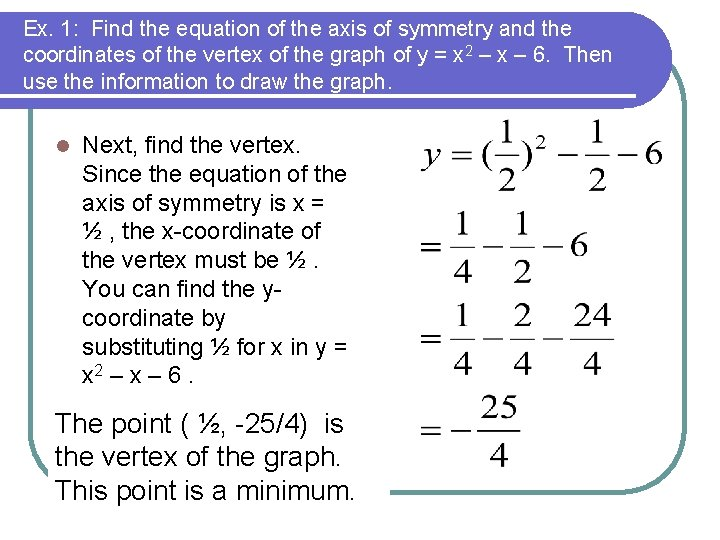Ex. 1: Find the equation of the axis of symmetry and the coordinates of the vertex of the graph of y = x 2 – x – 6. Then use the information to draw the graph. l Next, find the vertex. Since the equation of the axis of symmetry is x = ½ , the x-coordinate of the vertex must be ½. You can find the ycoordinate by substituting ½ for x in y = x 2 – x – 6. The point ( ½, -25/4) is the vertex of the graph. This point is a minimum.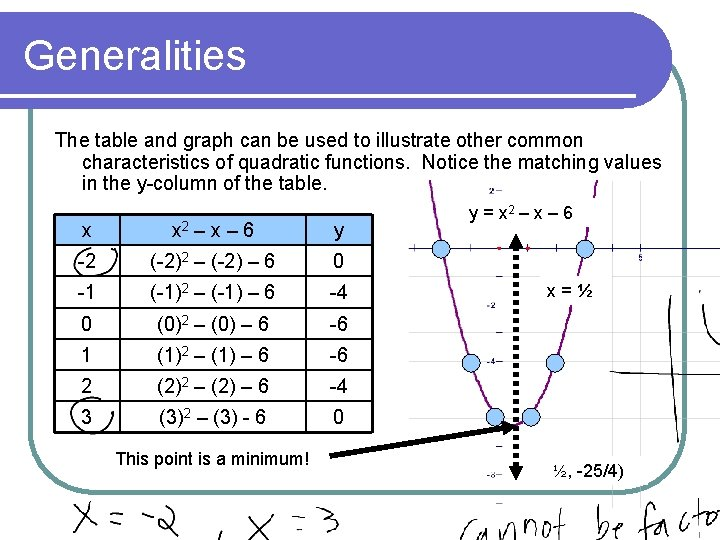Generalities The table and graph can be used to illustrate other common characteristics of quadratic functions. Notice the matching values in the y-column of the table. x x 2 – x – 6 y -2 (-2)2 – (-2) – 6 0 -1 (-1)2 – (-1) – 6 -4 0 (0)2 – (0) – 6 -6 1 (1)2 – (1) – 6 -6 2 (2)2 – (2) – 6 -4 3 (3)2 – (3) - 6 0 This point is a minimum! y = x 2 – x – 6 x=½ ½, -25/4)Solving Quadratic Equations Graphically SOLVING QUADRATIC EQUATIONS USING GRAPHS The solution of a quadratic equation in one variable x can be solved or checked graphically with the following steps: STEP 1 Write the equation in the form ax 2 + bx + c = 0. STEP 2 Write the related function y = ax 2 + bx + c. STEP 3 Sketch the graph of the function y = ax 2 + bx + c. The solutions, or roots, of ax 2 + bx + c = 0 are the x-intercepts.Checking a Solution Using a Graph Solve 1 2 x = 8 algebraically. Check your solution graphically. 2 SOLUTION 1 2 x = 8 2 CHECK Write original equation. x 2 = 16 Multiply each side by 2. x= 4 Find the square root of each side. Check these solutions using a graph.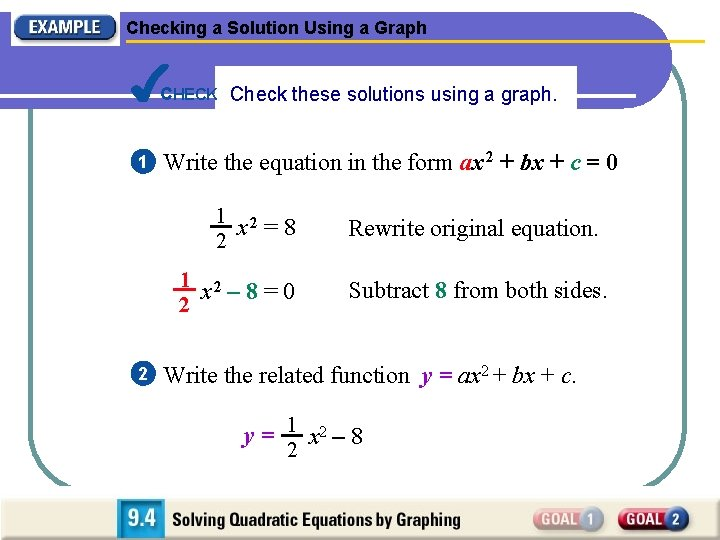Checking a Solution Using a Graph CHECK Check these solutions using a graph. 1 Write the equation in the form ax 2 + bx + c = 0 1 2 x =8 2 1 2 x – 8=0 2 2 Rewrite original equation. Subtract 8 from both sides. Write the related function y = ax 2 + bx + c. y = 1 x 2 – 8 2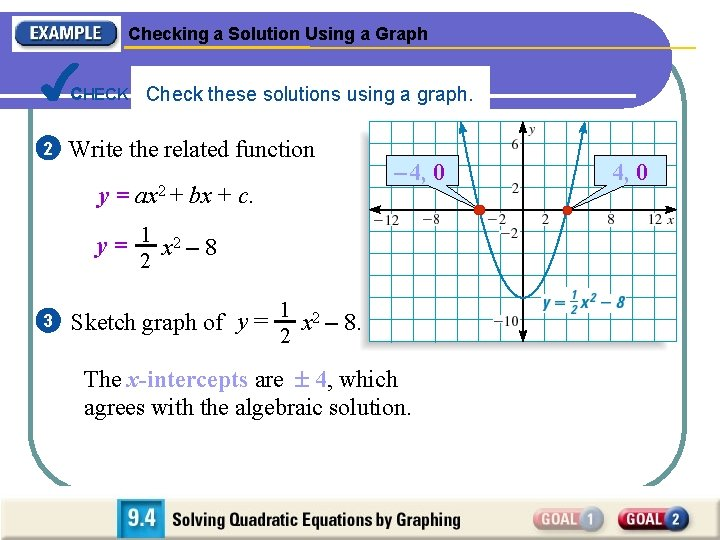Checking a Solution Using a Graph CHECK Check these solutions using a graph. 2 Write the related function y = ax 2 + bx + c. – 4, 0 y = 1 x 2 – 8 2 3 1 Sketch graph of y = x 2 – 8. 2 The x-intercepts are 4, which agrees with the algebraic solution. 4, 0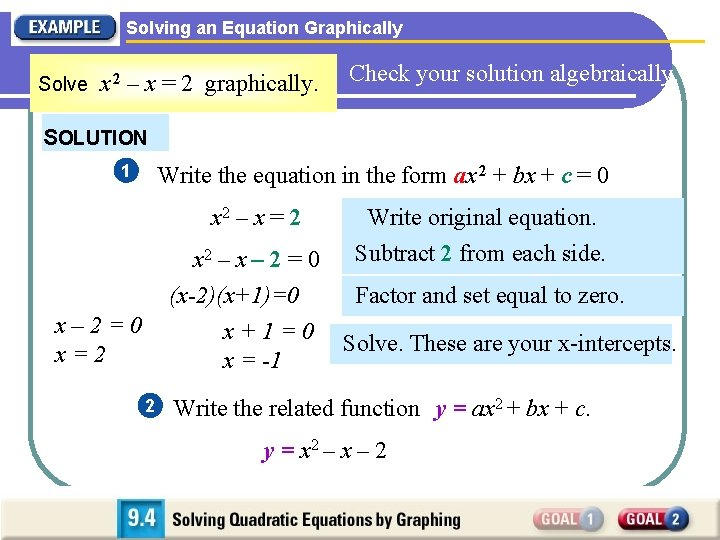Solving an Equation Graphically Solve x 2 – x = 2 graphically. Check your solution algebraically. SOLUTION 1 Write the equation in the form ax 2 + bx + c = 0 x 2 – x = 2 Write original equation. Subtract 2 from each side. x 2 – x – 2 = 0 (x-2)(x+1)=0 Factor and set equal to zero. x + 1 = 0 Solve. These are your x-intercepts. x = -1 x– 2=0 x=2 2 Write the related function y = ax 2 + bx + c. y = x 2 – x – 2Solving an Equation Graphically 2 Write the related function y = ax 2 + bx + c. y = x 2 – x – 2 – 1, 0 3 Sketch the graph of the function y = x 2 – x – 2 From the graph, the x-intercepts appear to be x = – 1 and x = 2. 2, 0Solving an Equation Graphically From the graph, the x-intercepts appear to be x = – 1 and x = 2. – 1, 0 CHECK You can check this by substitution. Check x = – 1: Check x = 2: x 2 – x = 2 ? ? (– 1) = 2 22 – 2 = 2 1+1=2 4– 2=2 (– 1) 2 – 2, 0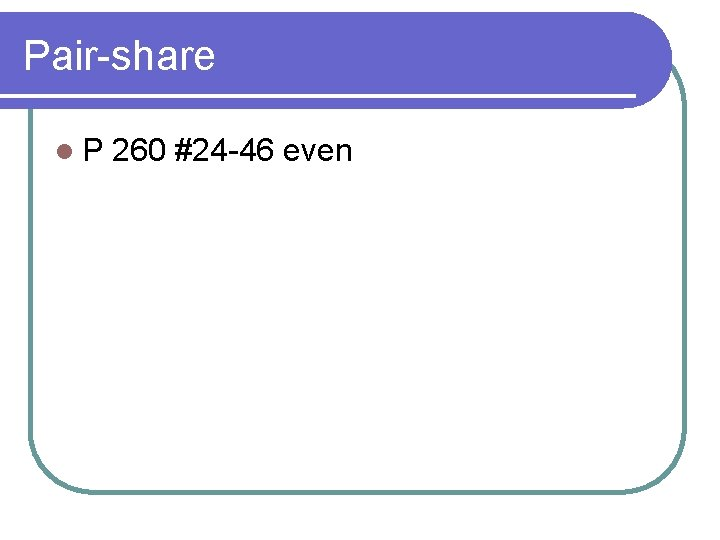Pair-share l. P 260 #24 -46 even